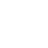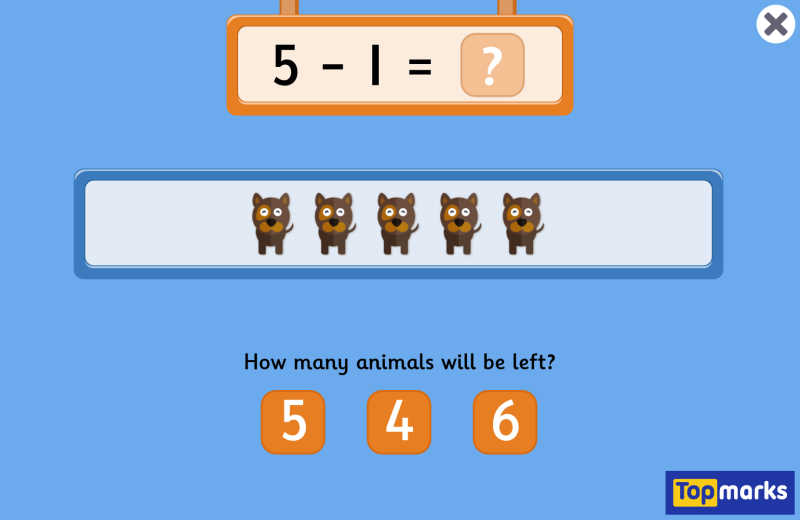# Subtraction To 10

1## QR CODE

Scan this on mobiles and tablets to quickly open this web page.This Subtraction to 10 game provides a simple introduction to the concept of subtraction. Each calculation features a subtraction within 10, along with a pictorial representation of the start number. Children can then proceed to subtract the required number from the row of animals. As they solve each calculation the remaining animals move to a number line and those animals which have been subtracted are crossed out. This visual representation of the subtraction process can help to reinforce the concept.

The game works well on an interactive whiteboard in a whole class or group situation.

AGE
5-6 Years
SUBJECTS
Maths Subtraction